# Perfect number facts for kids

Kids Encyclopedia Facts

A number is called a perfect number if by adding all the positive divisors of the number (except itself), the result is the number itself.

6 is the first perfect number. Its divisors (other than the number itself: 6) are 1, 2, and 3 and 1 + 2 + 3 equals 6. Other perfect numbers include 28, 496 and 8128.

## Perfect numbers that are even

Euclid discovered that the first four perfect numbers are generated by the formula 2n-1(2n - 1):

for n = 2: 21(22 - 1) = 6
for n = 3: 22(23 - 1) = 28
for n = 5: 24(25 - 1) = 496
for n = 7: 26(27 - 1) = 8128

Euclid saw that 2n - 1 is a prime number in these four cases. He then proved that the formula 2n-1(2n - 1) gives an even perfect number whenever 2n - 1 is prime (Euclid, Prop. IX.36).

Ancient mathematicians made many assumptions about perfect numbers based on the four they knew. Most of the assumptions were wrong. One of these assumptions was that since 2, 3, 5, and 7 are precisely the first four primes, the fifth perfect number would be obtained when n = 11, the fifth prime. However, 211 - 1 = 2047 = 23 × 89 is not prime and therefore n = 11 does not give a perfect number. Two other wrong assumptions were:

• The fifth perfect number would have five digits since the first four had 1, 2, 3, and 4 digits respectively.
• The perfect numbers would alternately end in 6 or 8.

The fifth perfect number (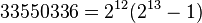$33550336=2^{12}(2^{13}-1)$) has 8 digits. This falsifies the first assumption. For the second assumption, the fifth perfect number indeed ends with a 6. However, the sixth (8 589 869 056) also ends in a 6. It is straightforward to show the last digit of any even perfect number must be 6 or 8.

In order for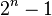$2^n-1$ to be prime, it is necessary that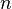$n$ should be prime. Prime numbers of the form 2n - 1 are known as Mersenne primes, after the seventeenth-century monk Marin Mersenne, who studied number theory and perfect numbers.

Two millennia after Euclid, Euler proved that the formula 2n-1(2n - 1) will yield all the even perfect numbers. Therefore, every Mersenne prime will yield a distinct even perfect number–there is a concrete one-to-one association between even perfect numbers and Mersenne primes. This result is often referred to as the "Euclid-Euler Theorem". Till January 2013, only 48 Mersenne primes are known. This means there are 48 perfect numbers known, the largest being 257,885,160 × (257,885,161 - 1) with 34,850,340 digits.

The first 42 even perfect numbers are 2n-1(2n - 1) for

n = 2, 3, 5, 7, 13, 17, 19, 31, 61, 89, 107, 127, 521, 607, 1279, 2203, 2281, 3217, 4253, 4423, 9689, 9941, 11213, 19937, 21701, 23209, 44497, 86243, 110503, 132049, 216091, 756839, 859433, 1257787, 1398269, 2976221, 3021377, 6972593, 13466917, 20996011, 24036583, 25964951 (sequence A000043 in OEIS)

The other 7 known are for n = 30402457, 32582657, 37156667, 42643801, 43112609, 57885161, 74207281. It is currently not known whether there are others between them.

It is still not known if there are infinitely many Mersenne primes and perfect numbers. The search for new Mersenne primes is the goal of the GIMPS distributed computing project.

Since any even perfect number has the form 2n-1(2n - 1), it is a triangular number, and, like all triangular numbers, it is the sum of all natural numbers up to a certain point; in this case: 2n - 1. Also, any even perfect number except the first one is the sum of the first 2(n-1)/2 odd cubes: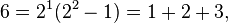$6 = 2^1(2^2-1) = 1+2+3, \,$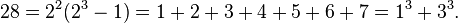$28 = 2^2(2^3-1) = 1+2+3+4+5+6+7 = 1^3+3^3.$

## Perfect numbers that are odd

It is not known whether there are any odd perfect numbers. Various results have been obtained, but none that has helped to locate one or otherwise resolve the question of their existence. Carl Pomerance has presented a heuristic argument which suggests that no odd perfect numbers exist. Also, it has been conjectured that there are no odd Ore's harmonic numbers. If true, this would mean that there are no odd perfect numbers.

Any odd perfect number N must satisfy the following conditions:

• N > 10300. It is likely that, in the near future, it will be proven that N > 10500. 
• N is of the form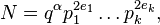$N=q^{\alpha} p_1^{2e_1} \ldots p_k^{2e_k},$
where:
• q, p1, ..., pk are distinct primes.
• q ≡ α ≡ 1 (modulo 4) (Euler).

### Proof

Let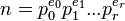$n=p_0^{e_0} p_1^{e_1} ...p_r^{e_r}$ be odd perfect number. Since divisor function is multiplicative,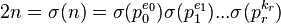$2n=\sigma(n)=\sigma(p_0^{e_0})\sigma(p_1^{e_1})...\sigma(p_r^{k_r})$.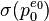$\sigma(p_0^{e_0})$ must be an even not divisible by 4 and all the remaining must be odd.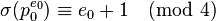$\sigma(p_0^{e_0}) \equiv e_0 + 1 \pmod {4}$ forces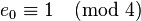$e_0 \equiv 1 \pmod {4}$.

• Either qα > 1020, or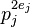$p_j^{2e_j}$ > 1020 for some j (Cohen 1987).
• N <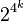$2^{4^{k}}$ (Nielsen 2003).
• The relation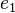$e_1$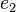$e_2$...≡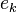$e_k$ ≡ 1 (modulo 3) is not satisfied (McDaniel 1970).
• The smallest prime factor of N is less than (2k + 8) / 3 (Grün 1952).
• The largest prime factor of N is greater than 108 (Takeshi Goto and Yasuo Ohno, 2006).
• The second largest prime factor is greater than 104, and the third largest prime factor is greater than 100 (Iannucci 1999, 2000).
• N has at least 75 prime factors; and at least 9 distinct prime factors. If 3 is not one of the factors of N, then N has at least 12 distinct prime factors (Nielsen 2006; Kevin Hare 2005).

## Minor results

Even perfect numbers have a very precise form; odd perfect numbers are rare, if indeed they do exist. There are a number of results on perfect numbers that are actually quite easy to prove but nevertheless superficially impressive; some of them also come under Richard Guy's Strong Law of Small Numbers:

• Every odd perfect number is of the form 12m + 1 or 4356m + 1089 or 468m + 117 or 2916m + 729 (Roberts 2008).
• An odd perfect number is not divisible by 105 (Kühnel 1949).
• Every odd perfect number is the sum of two squares (Stuyvaert 1896).
• A Fermat number cannot be a perfect number (Luca 2000).
• The only even perfect number of the form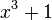$x^3+1$ is 28 (Makowski 1962).
• By dividing the definition through by the perfect number N, the reciprocals of the factors of a perfect number N must add up to 2:
• For 6, we have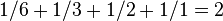$1/6 + 1/3 + 1/2+ 1/1 = 2$;
• For 28, we have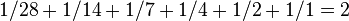$1/28 + 1/14 + 1/7 + 1/4 + 1/2 + 1/1 = 2$, etc.
• The number of divisors of a perfect number (whether even or odd) must be even, since N cannot be a perfect square.
• From these two results it follows that every perfect number is an Ore's harmonic number.

## Related concepts

The sum of proper divisors gives various other kinds of numbers. Numbers where the sum is less than the number itself are called deficient, and where it is greater than the number, abundant. These terms, together with perfect itself, come from Greek numerology. A pair of numbers which are the sum of each other's proper divisors are called amicable, and larger cycles of numbers are called sociable. A positive integer such that every smaller positive integer is a sum of distinct divisors of it is a practical number.

By definition, a perfect number is a fixed point of the restricted sum-of-divisors function s(n) = σ(n) − n, and the aliquot sequence associated with a perfect number is a constant sequence.Perfect number Facts for Kids. Kiddle Encyclopedia.